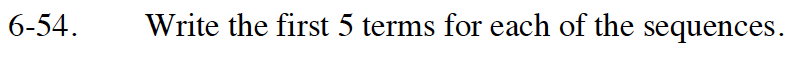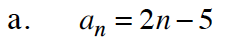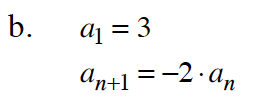Home > GB8I > Chapter cc46 > Lesson cc46.2.1 > Problem6-54

6-54.
1. Write the first 5 terms for each of the sequences. Homework Help ✎

1. an = 2n – 5

2. a1 = 3
an+1 = –2 · ana1 = 2(1) − 5
a2 = 2(2) − 5Note that a1 is given.

a2 : a1+1 = −2 · a1
a2 = −2(3)

a3 : a2+1 = −2 · a2
a3 = −2(−6)